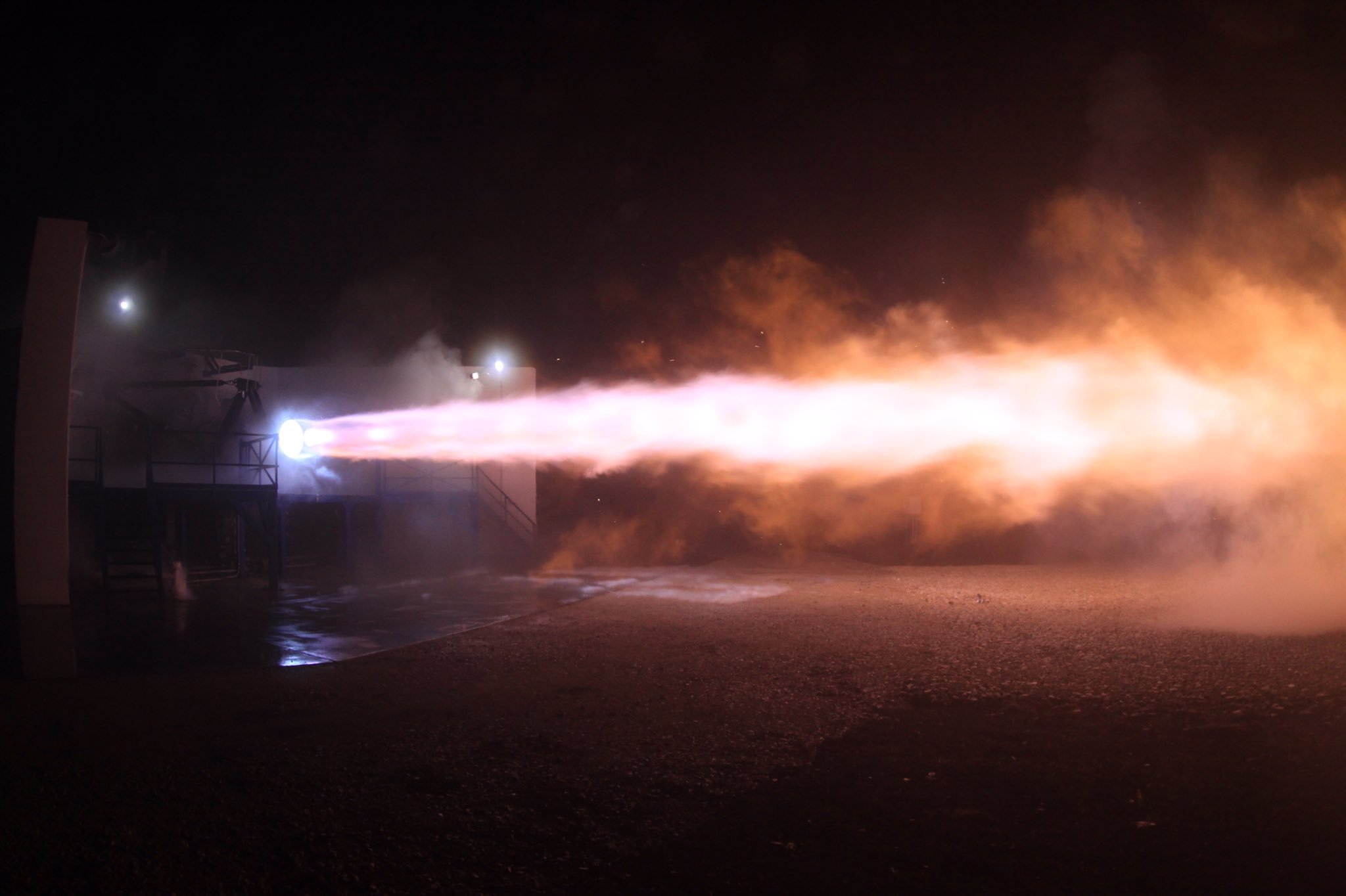# Combustion Jet

The equation of motion for rockets is $m_\textrm{r}\,\dot{v}_\textrm{r}= u_\textrm{e}\, \dot{m}_\textrm{r},$ where $u_\textrm{e}$ is the speed of the exhaust shooting out the back. The final velocity of the rocket is a few multiples of the exhaust speed, so it's quite important, but in physics textbooks, $u_\textrm{e}$ is usually treated like a freely adjustable parameter whose origin and precise value is a detail left for engineers to worry about. With a little bit of approximation, we can do better than that.

Estimate $u_\textrm{e}$ for the combustion of methane $(\ce{CH4}),$ the propellant used by SpaceX for its Raptor rocket engine program.Details and Assumptions:

• The specific enthalpy of combustion for methane is $\Delta_c H \approx \SI[per-mode=symbol]{890}{\kilo\joule\per\mole}.$
• Assume that all the energy released in combustion goes into the kinetic energy of the gas.
• Beyond its performance, methane is nice because it could potentially be produced on other planets via the Sabatier reaction $\ce{CO2 + 4H2 -> CH4 + 2H2O}.$
×